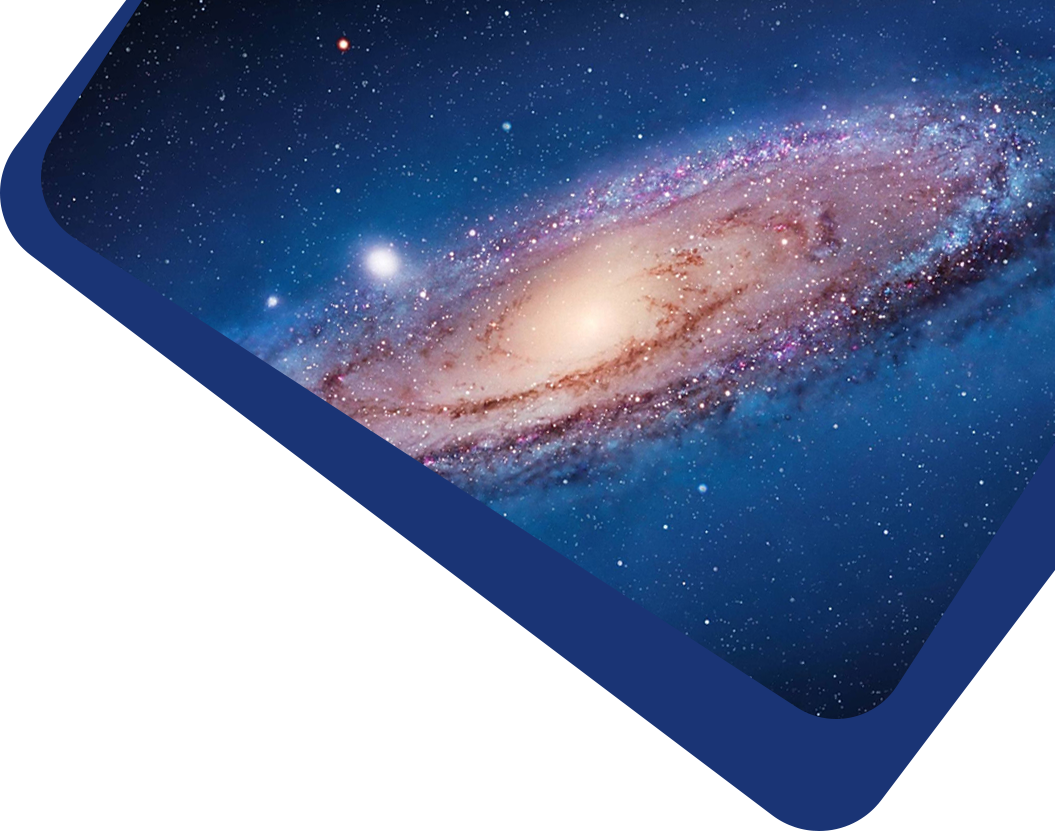Vol 21, No 4

## Explaining recently studied intermediate luminosity optical transients (ILOTs) with jet powering

#### Noam Soker, Noa Kaplan

Abstract

Abstract We apply the jet-powered ILOT scenario to two recently studied intermediate luminosity optical transients (ILOTs), and find the relevant shell mass and jets’ energy that might account for the outbursts of these ILOTs. In the jet-powered ILOT scenario, an accretion disk around one of the stars of a binary system launches jets. The interaction of the jets with a previously ejected slow shell converts kinetic energy to thermal energy, part of which is radiated away. We apply two models of the jet-powered ILOT scenario. In the spherical shell model, the jets accelerate a spherical shell, while in the cocoon toy model the jets penetrate into the shell and inflate hot bubbles, the cocoons. We find consistent results. For the ILOT (ILRT: intermediate luminosity red transient) SNhunt120 we find the shell mass and jets’ energy to be $$M_{\rm s} \simeq 0.5 − 1 M_{\odot}$$ and $$E_{2j}\simeq 5 \times 10^{47}\rm erg$$, respectively. The jets’ half opening angle is $$\alpha_j\simeq 30^{\circ}- 60^{\circ}$$. For the second peak of the ILOT (luminous red nova) AT 2014ej we find these quantities to be $$M_{\rm s}\simeq 1-2 M_{\odot}$$ and $$E_{2j}\simeq 1.5 \times 10^{48}\rm erg$$, with $$\alpha_j\simeq 20^{\circ} - 30^{\circ}$$. The models cannot tell whether these ILOTs were powered by a stellar merger that leaves one star, or by mass transfer where both stars survived. In both cases the masses of the shells and energies of the jets suggest that the binary progenitor system was massive, with a combined mass of $$M_1 + M_2 \geq 10 M_{\odot}$$.

Keywords

Keywords binaries: close — stars: jets — stars: variables: general

Full Text
Refbacks

• There are currently no refbacks.